Courses

# Test: Linear Vibration Analysis - 2

## 10 Questions MCQ Test Theory of Machines (TOM) | Test: Linear Vibration Analysis - 2

Description
This mock test of Test: Linear Vibration Analysis - 2 for Mechanical Engineering helps you for every Mechanical Engineering entrance exam. This contains 10 Multiple Choice Questions for Mechanical Engineering Test: Linear Vibration Analysis - 2 (mcq) to study with solutions a complete question bank. The solved questions answers in this Test: Linear Vibration Analysis - 2 quiz give you a good mix of easy questions and tough questions. Mechanical Engineering students definitely take this Test: Linear Vibration Analysis - 2 exercise for a better result in the exam. You can find other Test: Linear Vibration Analysis - 2 extra questions, long questions & short questions for Mechanical Engineering on EduRev as well by searching above.
QUESTION: 1

### All of the following statements are correct, except,

Solution:

Mass does not depend on altitude, so natural frequency remains unaffected.

QUESTION: 2

### The critical speed of the shaft depends upon

Solution:

Critical speed of a shaft is nothing but its natural frequency and it iswhere,
K = stiffness of the shaft.
m = mass of the shaft

QUESTION: 3

### The equation: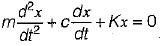represents

Solution:

Since excitation force is absent in the equation hence it is the equation of free, damped vibration.

QUESTION: 4

The governing equation of a specific simple vibrating system is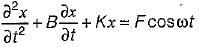The system is subjected to

Solution:

Since excitation force is present in the equation hence it is the equation of forced vibration.

QUESTION: 5

The static deflection of a shaft under a flywheel is 4 mm. Take g = 10 m/s2. What is the critical speed in rad/s?

Solution: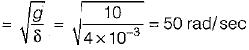QUESTION: 6

A flexible rotor-shaft system comprises of a 10 kg rotor disc placed in the middle of a massless shaft of diameter 30 mm and length 500 mm between bearings (shaft is being taken massless as the equivalent mass of the shaft is included in the rotor mass) mounted at the ends. The shaft is made of steel for which the value of E is 2.1 x 1011 Pa. What is the critical speed of rotation of the shaft.

Solution:

For shaft loaded at middle with mass M
Deflection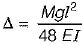Moment of inertia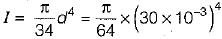So deflection of shaft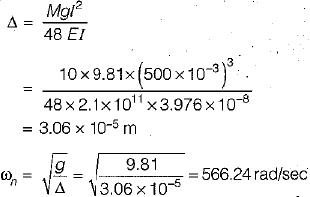QUESTION: 7

When a body is subjected ,to transverse vibration, the stress induced in the body will be

Solution:

In case of transverse vibration, bending stresses are generated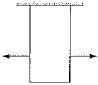QUESTION: 8

Whirling speed of a shaft coincides with the natural frequency of its

Solution:

Whirling of shaft occurs when the natural frequency of transverse vibration matches with the frequency of rotating shaft.

QUESTION: 9

For given figure shows a spring mass system where the mass m is fixed in between two springs of stiffness s1 and s2. What is the equivalent spring stiffness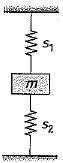Solution:

Since the springs are connected in parallel
seq = s1 + s2

QUESTION: 10

The rate of decay of oscillations is known as

Solution:

Natural logarithm of the amplitude reduction factor is known as logarithmic decrement.